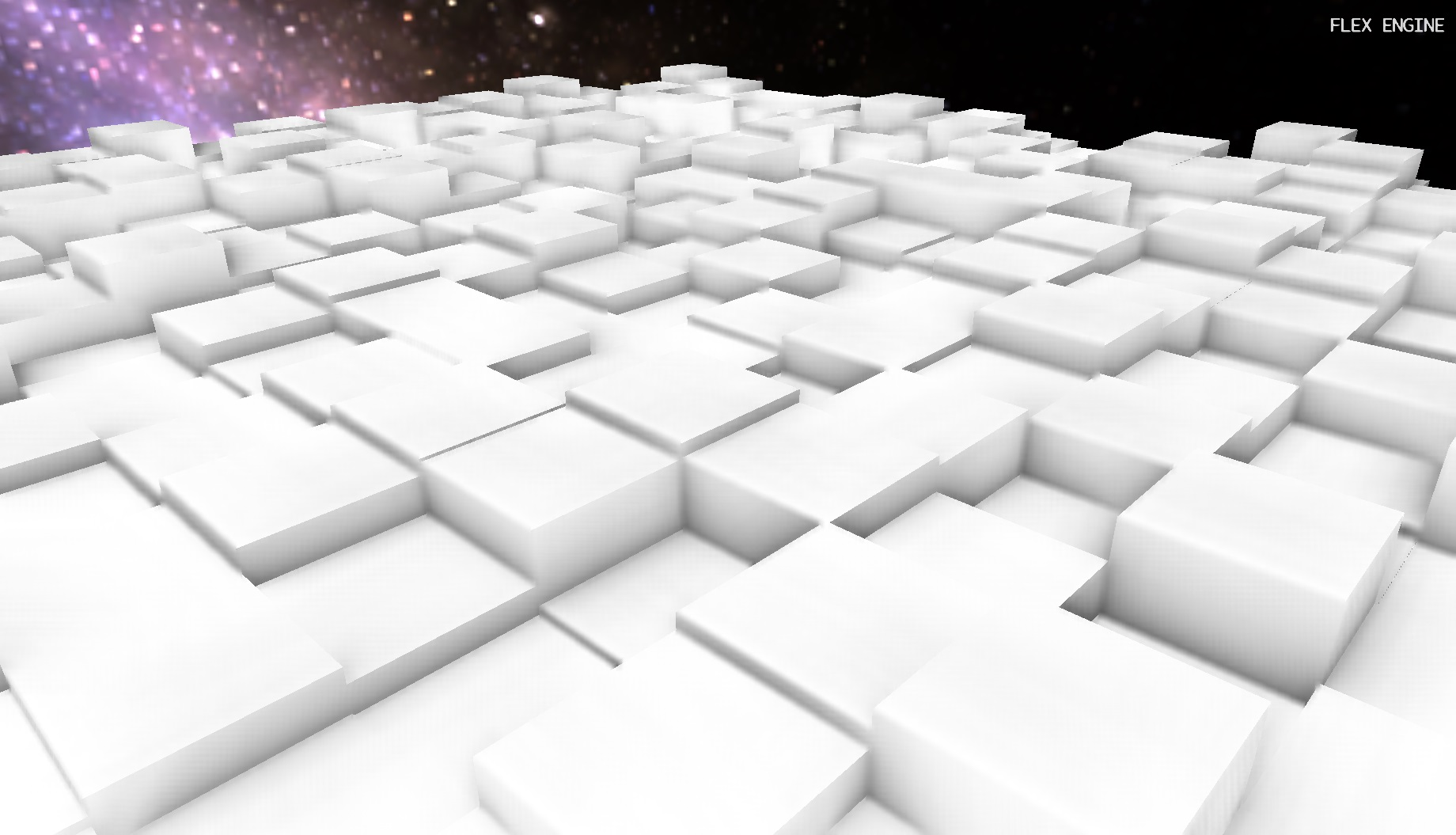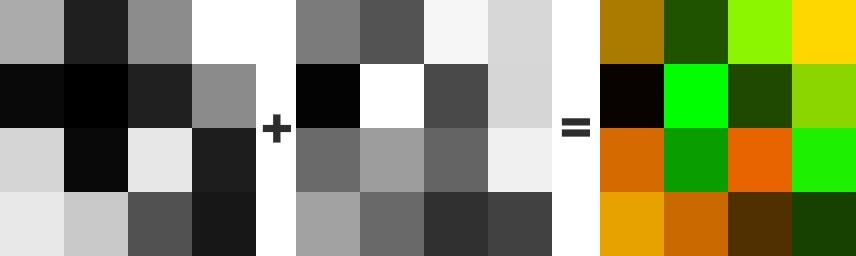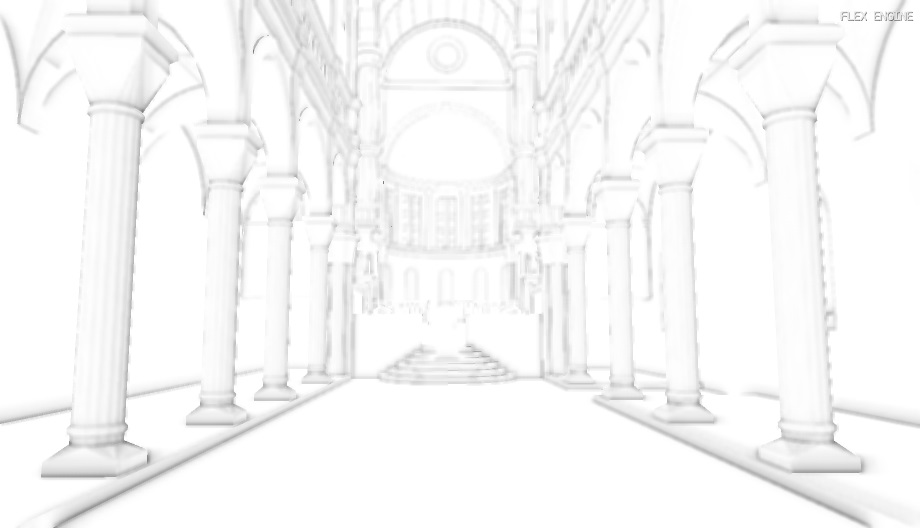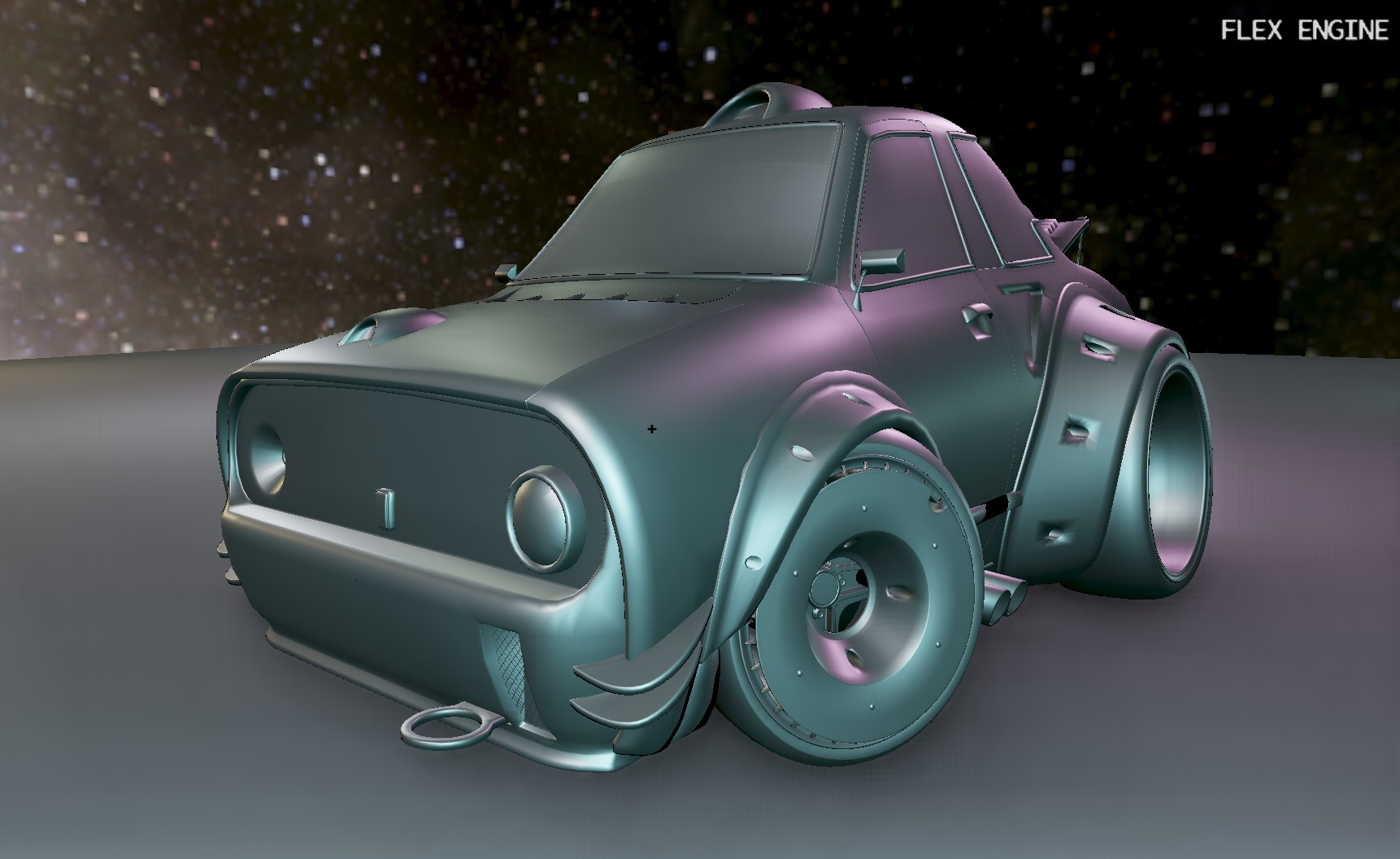SSAO (Screen-space ambient occlusion) is a widespread technique employed by many games to simulate the shadowing effect of objects occluding other nearby objects. It was originally proposed by Crytek in 2007 (original paper ), and has since seen many improvements. I’ve implemented a variant of it in Flex recently, and this is a short discussion of the implementation details.

The necessary inputs are the scene depth and normals, both in view space. The depth buffer will be used as a rough estimate of how occluded each pixel is, and the normals will be used to orient a hemisphere about each pixel to generate our sample points in. Because Flex already supports deferred rendering, these two input buffers were readily available. The only change necessary was to output the normals in view space, rather than world space.

Instead of depth, some implementations require a three-channel position buffer as input. I’ve opted to reconstruct position from the depth buffer instead to save on texture bandwidth. There’s a number of ways to do this, if you’re implementing this yourself then definitely give this three part series by MJP a read. I opted for using the already existing view-space hardware depth buffer. Here’s how I’m unpacking that into a view-space position:

``````vec3 reconstructVSPosFromDepth(vec2 uv)
{
float depth = texture(in_Depth, uv).r;
float x = uv.x * 2.0 - 1.0;
float y = (1.0 - uv.y) * 2.0 - 1.0;
vec4 pos = vec4(x, y, depth, 1.0);
vec4 posVS = uboConstant.invProj * pos;
return posVS.xyz / posVS.w;
}
``````

In order to avoid banding artifacts we will modulate the sample points by some random noise. A 4x4 noise texture can be generated at startup. To get random rotations around the z-axis, our texture will contain values in the range [-1.0, 1.0] in the red and green channels. This requires the use of a floating point texture format, but could easily be scaled and biased to fit into an integral format just as well.

Packed two channel noise textureTo orient the hemisphere about the normal, I first use the Gram-Schmidt process to obtain a local coordinate frame:

``````ivec2 depthSize = textureSize(in_Depth, 0);
ivec2 noiseSize = textureSize(in_Noise, 0);
float renderScale = 0.5; // SSAO is rendered at 0.5x scale
vec2 noiseUV = vec2(float(depthSize.x)/float(noiseSize.x),
float(depthSize.y)/float(noiseSize.y))
* ex_UV * renderScale;
vec3 randVec = texture(in_Noise, noiseUV).xyz;

vec3 tangent = normalize(randVec - normal * dot(randVec, normal));
vec3 bitangent = cross(tangent, normal);
mat3 TBN = mat3(tangent, bitangent, normal);
``````

Then the real meat of the algorithm can commence:

``````float bias = 0.01;

float occlusion = 0.0;
for (int i = 0; i < SSAO_KERNEL_SIZE; i++)
{
vec3 samplePos = TBN * uboConstant.samples[i].xyz;
samplePos = posVS + samplePos * SSAO_RADIUS;

// Convert view-space position into clip-space
vec4 offset = vec4(samplePos, 1.0);
offset = uboConstant.projection * offset;
offset.xy /= offset.w;
offset.xy = offset.xy * 0.5 + 0.5;
offset.y = 1.0 - offset.y;

vec3 reconstructedPos = reconstructVSPosFromDepth(offset.xy);
occlusion += (reconstructedPos.z <= samplePos.z-bias ? 1.0 : 0.0);
}
occlusion = 1.0 - (occlusion / float(SSAO_KERNEL_SIZE));

fragColor = occlusion;
``````

In essence, we count all points that are farther into the scene than the current fragment as occluders, and all others as non-occluders. Note that this is calculated at half-size since it a low-frequency effect and this quarters the execution time necessary.

This solution however has some gaping holes which need to be patched up. For one, large depth discontinuities are ignored, causing a dark halo effect around objects which are well in front of other objects. This can be addressed by scaling the occlusion factor based a points distance from the center.

Another big issue with the implementation thus far is the level of noise. To achieve an acceptable result, we need to apply a blur. The blur should run at full-resolution in order to avoid cracks at the edges of objects. I first implemented a simple box blur, but later replaced this with a two-pass edge-preserving bilateral blur covered in more detail below.

``````float rangeCheck = smoothstep(0.0, 1.0, uboConstant.ssaoRadius / abs(reconstructedPos.z - samplePos.z - bias));
occlusion += (reconstructedPos.z <= samplePos.z - bias ? 1.0 : 0.0) * rangeCheck;
``````### Two-pass bilateral blur

An NxN box blur is a simple effect to implement, however also an expensive one to compute, especially as N increases. A much more efficient approach is to use a separable blur. By splitting the blur into two passes, we can first calculate the horizontal blur using just N taps, and then the vertical blur in a second pass, taking another N taps. This produces the same image, but with only 2N taps rather than N2. The input, result after one pass, and final blurred result are shown below.When updating the blur to occur in two passes, I also passed along the normal and depth buffer to the blur shader in order to make the blur bilateral. When sampling neighbouring pixels, samples whose depth lie outside of a given distance or whose normal differs by a small threshold are ignored. This allows for edges to remain sharp, and depth discontinuities to be respected.

### Specialization Constants

I used Spir-V’s specialization constant feature to give my constant uniform kernel size a unique ID so it can be updated by the host. The SSAO graphics pipeline needs to be recreated for the change to take effect, but still this allows for tweaking in near real-time, with the performance benefits of a static value. See  for a great explanation.Thanks for reading! As always, the source code is all available on GitHub.

Thanks to luyssport for the car model!